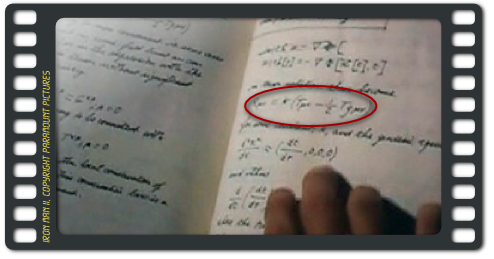Smarter than Tony Stark

 Well, I wish I were… Anyhoo, I went to the movies and saw Iron Man II a few hours ago. Slightly disappointing, maybe because I was expecting much from Don Cheadle and Mickey Rourke, and, well, because the french speaking scenes were so totally weird (I had no idea that people from Monaco were speaking with such a strong Québécois accent…).But that is not the point of this post. I saw the film through my geeky eyes, and I was stricken by a particular scene.

Okay, this is just a small detail that caught my attention. I’m weird. I know. I (kind of) assume it.

Usually, the laws of physics don’t apply in Hollywood movies, and especially in superhero movies, including this one. But, at one point, Tony Stark is given by Nick Fury a suitcase containing a few old films and notes from his father (Howard Stark)

Here’s a shot from the film, focusing on the notes :The circled formula is what caught my eyes and triggered an intense googling effort to find this shot and check if I was indeed crazy (or not). It reads as follows:$R_{\mu\nu} = K \left(T_{\mu\nu} - \frac{1}{2} T g_{\mu\nu}\right)$

which is accurate, as it is a “trace-reversed” form of actual Einstein’s field equations:$R_{\mu\nu} = \frac{8\pi G}{c^4} \left(T_{\mu\nu} - \frac{1}{2} T g_{\mu\nu}\right)+\Lambda g_{\mu\nu}$

where the Cosmological Constant ($\Lambda$) would have been omitted.

Usually, Einstein’s field equations are written under this form:$G_{\mu\nu} + \Lambda g_{\mu\nu} = \frac{8\pi G}{c^4} T_{\mu\nu}$ where$G_{\mu\nu} = R_{\mu\nu} - \frac{1}{2} Rg_{\mu\nu}$

or under this simpler and incredibly beautiful form (neglecting the cosmological constant):$G_{\mu\nu} = \frac{8\pi G}{c^4} T_{\mu\nu}$

The rest of the notes apparently deal with the conservation of energy-momentum$T^{ab};b = 0$ and other simple properties of General Relativity.

Whatever the meaning of all these equations is (got one post on General Relativity in preparation), what is truly remarkable is that:

• From what can be read, it seems to be accurate. These are quite probably extracts from a graduate student textbook on General Relativity
• The producers took the time to hire someone to write down actual physics, which is pretty cool
• The physics involved (gravitational field) has quite probably nothing to do with an Arc Reactor (whatever this could be)…

… and this very last point makes me think that I may be smarter than Tony Stark, who doesn’t seem to mind at all. Which is bad news for a genius, since it is elementary physics.

Now, since I have soooo brilliantly demonstrated that I am smarter than a super-genius, why isn’t there hot chicks dancing around in my apartment wearing iron-man suits/bikinis ? Come on, where are the Ironettes ?

Okay, I might not be as rich and attractive as Tony Stark 🙁

This site uses Akismet to reduce spam. Learn how your comment data is processed.